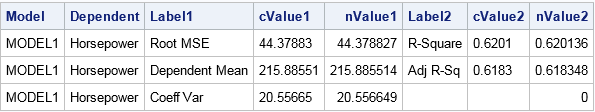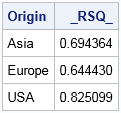In the beginning, SAS created procedures and output. The output was formless and void. Then SAS said, "Let there be ODS," and there was ODS. Customers saw that ODS was good, and SAS separated the computation from the display and management of output.

The preceding paragraph oversimplifies the SAS Output Delivery System (ODS), but the truth is that ODS is a powerful feature of SAS. You can use ODS to send SAS tables and graphics to various output destinations, including HTML, PDF, RTF, and PowerPoint. You can control the style and attributes of the output, thus creating a customized report. There have been hundreds of papers and books written about ODS. A very basic introduction is Olinger (2000) "ODS for Dummies."

To a statistical programmer, the most useful destination is the OUTPUT destination. The OUTPUT destination sends a table or graph to a SAS data set. Consequently, you can programmatically access each element of the output.

The implications of the previous statement are monumental. I cannot overstate the importance of the OUTPUT destination, so let me say it again:

The ODS OUTPUT destination enables you to store any value that is produced by any SAS procedure. You can then read that value by using a SAS program.

The ODS OUTPUT destination answers a common question that is asked by new programmers on SAS discussion forums: "How can I get a statistic into a data set or into a macro variable?" The steps are as follows:

1. Use ODS TRACE ON (or the SAS documentation) to find the name of the ODS table that contains the statistic that you want.
2. Use the ODS OUTPUT statement to specify the table name and a data set name. The syntax is ODS OUTPUT TableName=DataSetName. Then run the procedure to generate the table.
3. Read the data set to obtain the value of the statistic.

### Find the name of the ODS table

As an example, suppose that you intend to use PROC REG to perform a linear regression, and you want to capture the R-square value in a SAS data set. The documentation for the procedure lists all ODS tables that the procedure can create, or you can use the ODS TRACE ON statement to display the table names that are produced by PROC REG. The data are the 428 vehicles in the Sashelp.Cars data set, which is distributed with SAS:

```ods trace on; /* write ODS table names to log */ proc reg data=Sashelp.Cars plots=none; model Horsepower = EngineSize Weight; quit; ods trace off; /* stop writing to log */``````Output Added: ------------- Name: FitStatistics Label: Fit Statistics Template: Stat.REG.FitStatistics Path: Reg.MODEL1.Fit.Horsepower.FitStatistics -------------```

By looking at the output, you can see that the third table contains the R-square value. By looking at the SAS log, you can see that the name of the third table is "FitStatistics."

### Save the table to a SAS data set

Now that you know the name of the ODS table is "FitStatistics," use the ODS OUTPUT destination to write that table to a SAS data set, as follows:

```ods output FitStatistics=Output; /* the data set name is 'Output' */ proc reg data=Sashelp.Cars plots=none; /* same procedure call */ model Horsepower = EngineSize Weight; quit;   proc print data=Output noobs; run;```The output from PROC PRINT shows the structure of the output data set. Notice that the data set often looks different from the original displayed table. The data set contains non-printing columns (like Model and Dependent) that do not appear in the displayed table. The data set also contains columns that contain the raw numerical values and the (formatted) character values of the statistics. The columns cValue1 and nValue1 represent the same information, except that the cValue1 is a character column whereas nValue1 is a numerical column. The same applies to the cValue2 and nValue2 columns. The character values might contain formatted or rounded values.

### Read the value of the statistic into a macro variable

From the previous PROC PRINT output, you can see that the numerical value of the R-square statistic is in the first row and is in the nValue2 column. You can therefore read and process that value by using a standard WHERE clause. For example, the following statements use the SYMPUTX subroutine to create a macro variable that contains the value of the R-square statistic:

```data _null_; set Output; if Label2="R-Square" then call symputx("RSq", nValue2); run;   %put RSq = &RSq;```
`RSq = 0.6201360929`

The SAS log shows that the R-square value is now contained in the Rsq macro variable.

Storing the statistic in a macro variable is only one way to use the data set. You could also read the statistics into PROC IML or PROC SQL for further computation, or show the value of the statistic in a graph.

### BY-group processing: Multiple samples and multiple statistics

The previous sections show how to save a single table to a SAS data set. It is just as easy to create a data set that contains multiple statistics, one for each level in a BY-group analysis.

Suppose that you want to run several regressions, one for each value of the Origin variable, which has the values "Asia," "Europe," and "USA." The following call to PROC SORT sorts the data by the Origin variable. The sorted data are stored in the CARS data set.

```proc sort data=Sashelp.Cars out=Cars; by Origin; run;```

You can then specify Origin on the BY statement in PROC REG to carry out three regression analyses. When you run a BY-group analysis, you might not want to see all of the results displayed on the computer screen, especially if your goal is to save the results in an output data set. You can use the ODS EXCLUDE statement to suppress SAS output.

```ods exclude all; /* suppress tables to screen */ ods output FitStatistics=Output2; /* 'Output2' contains results for each BY group */ proc reg data=Cars plots=none; by Origin; model Horsepower = EngineSize Weight; quit; ods exclude none; /* no longer suppress tables */   proc print data=Output2 noobs; where Label2="R-Square"; var Origin Label2 nValue2; run;```The output from PROC PRINT shows the R-square statistics for each model. Notice that the BY-group variables (in this case, Origin) are added to output data sets when you run a BY-group analysis. You can now use the statistics in programs or graphs.

### Alternatives to using ODS OUTPUT

Some procedures provide an alternative option for creating an output data set that contains statistics. Always check the SAS documentation to see if the procedure provides an option that writes common statistics to an output data set. For example, the documentation for the PROC REG statement states that you can use the OUTEST= option with the RSQUARE option to obtain an output data set that contains the parameter estimates and other model statistics such as the R-square value. Thus for this example, you do not need to use the ODS OUTPUT statement to direct the FitStatistics table to a data set. Instead, you can obtain the statistic as follows:

```proc reg data=Cars NOPRINT outest=Output3 RSQUARE; /* statistics in 'Output3' */ by Origin; model Horsepower = EngineSize Weight; quit;   proc print data=Output3 noobs; format _RSQ_ 8.6; var Origin _RSQ_; run;```### Summary

In summary, the ODS OUTPUT statement enables you to create a data set that contains any statistic that is produced by a SAS procedure. You can use the ODS OUTPUT statement to capture a statistic and use it later in your program.

ShareDistinguished Researcher in Computational Statistics

Rick Wicklin, PhD, is a distinguished researcher in computational statistics at SAS and is a principal developer of SAS/IML software. His areas of expertise include computational statistics, simulation, statistical graphics, and modern methods in statistical data analysis. Rick is author of the books Statistical Programming with SAS/IML Software and Simulating Data with SAS.

1.Prasanna Sondur on

Well explained. Necessary and very useful info.Thanks for sharing.

2.I was aware that you could just use the outest= option for proc reg, but didn't know about ODS output. Do you happen to know if the name of the table is specific to each procedure? For example here you mention that the proc reg gives the table name as 'FitStatistics' - would it be different for if you did for example a proc ttest? Similarly for if you did more than one proc reg, would it increment the names to for example FitStatistics2, FitStatistics3, etc.?

Was a good post, thanks for sharing :)

3.Very helpful post--thanks. One small addition is that the output label can be used in quotes if that's clearer than the name. For example, the following statements give the same output from PROC MIXED:

ods output "Solution for Fixed Effects"=ods_label;
ods output SolutionF=ods_name;

4.This was a powerful feature I learned from the SAS Press book ODS the Basics by Lauren Haworth Lake which became the book ODS the Basics and Beyond https://www.sas.com/store/books/categories/examples/output-delivery-system-the-basics-and-beyond/prodBK_61686_en.html . This is great tool in the tool box. I tend to just use it for PROC FREQ output but know that it can work with most any PROC.

5.Peter Lancashire on

Thanks for explaining what happens in BY-group processing. I have sent some feedback to the documentation people asking them to add this explicitly to the ODS OUTPUT page with an example showing TWO BY-group variables (so we know it works for more than one).

6.Hi,I'm a beginner user at SAS. I'm comparing 3 groups scores with using npar1way VW procedure on simulation data. I'm trying to calculate type 1 error using p values. I want to make a table that gives computed type I error rate from the comparison repeat at 50 times. How can I get output as a single table.

•I suggest you post your question and code to the SAS Support Communities. For this question, probably the Statistical Procedures Community is best.

7.This was a great article! One question, I would normally have put the ODS OUTPUT inside a procedure, but I notice you and others put it before the PROC call, does it make a difference performance wise or in any other way?

•8.Dmitrii Desiatkov on

Great Tip. Saved a lot of headache.

9.The variables label and variables format in the output files are not the same when using ods output or older syntax.
I'm not aware of any option which would allow to remove any format/label from the output dataset.

•> The variables label and variables format in the output files are not the same when using ods output or older syntax.

That is a true statement.

> I'm not aware of any option which would allow to remove any format/label from the output dataset.

You can use PROC DATASETS to remove attributes, as shown below:

```proc reg data=sashelp.class plots=none; model weight = height age; ods output ParameterEstimates=PE; quit; proc contents data=PE; ods select Variables; run;   proc datasets lib=work nolist; modify PE; format _all_; /* or specify the names of the variables */ attrib _all_ label=''; run; proc contents data=PE; ods select Variables; run;```